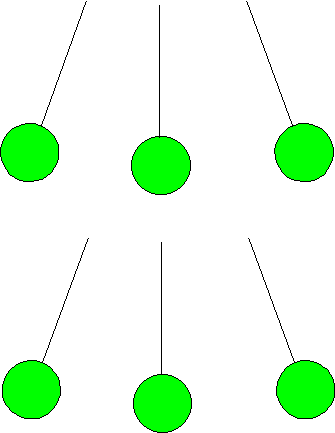BACK

Quiz on Newton's Laws

(Note: these questions were all taken from old 109N homeworks on the web.)

1. State Newton's Second Law.

Suppose you are standing on a spring bathroom scale in an elevator which is at rest. What is your acceleration? What forces are acting on you? What is the reading on the bathroom scale?

Suppose now you push the button for a higher floor, and the elevator begins to move. After three seconds it is going up at a steady one meter per second. Did you accelerate? Are you still accelerating? How do you think the reading on the scale will vary as the elevator begins to move then settles to a steady speed? (Just answer qualitatively - no numbers expected.)

Now imagine disaster - the rope holding the elevator snaps, there are no safety features, and the elevator plunges down the shaft in free fall. You avail yourself of this golden opportunity to check Newton's Laws further, since it is unlikely to be repeated, at least for you personally. You stay calmly on the spring scale. What does it read?

2. Sketch an elliptical orbit for a planet going around a sun. At the point in the orbit where the planet is closest to the sun, draw an arrow indicating the velocity of the planet as it goes around the orbit, and another arrow showing its acceleration. (The important point here is to get the directions right).

Now draw arrows indicating velocity and acceleration at the planet's furthest point from the sun.

Finally, draw velocity and acceleration arrows at a point in between.

(I want to see that you have some rough idea how the velocity and acceleration vary on going around the elliptical orbit).

3. Newton's Second law states that the acceleration of a body is proportional to the total external force acting on the body.

Suppose you are standing still, then begin to walk. What external force caused your acceleration?

Suppose you are standing still, then jump. What external force caused your acceleration?

4. Straight line motion: sketch qualitatively how downward acceleration varies with time for:

(a) a sky diver, from plane to ground,

(b) a bungee jumper, from start to finish.

For purposes of this question, assume survival of both the above.5.(a) The figure represents a pendulum (a bob -- a small round weight, on a string) swinging back and forth, assume it is shown at the furthest point of its swing in each direction and in the middle of the swing. On the first set, show the velocity vector appropriate to these positions in the swing, on the lower set of pictures show the acceleration vectors for the three instants. Don't worry too much about representing the magnitudes, but think carefully about direction.

(b) Suppose the string is cut as the pendulum swings through the lowest point. Sketch the path of the pendulum from before the swing is cut until well after. How does the velocity of the pendulum the instant before the string is cut compare with that the instant after? How does the acceleration of the pendulum the instant before the string is cut compare with that the instant after?

(c) Now suppose instead the string is cut when the pendulum has reached its furthest point to the right in the swing. Sketch the path of the pendulum from before the string is cut until well after, and again compare the velocity and acceleration of the pendulum the instant before the string is cut with the instant after.

6. (a) Suppose you throw a tennis ball at 45 degrees to the horizontal and it stays in the air four seconds. Ignoring air resistance, how far away from you does it land?

(Neglect the height of your hand above ground.) What was its speed on leaving your hand?

(b) Same as (a), except now it stays in the air only two seconds. How far did you throw it? How fast did you throw it? Now use a real ball -- estimate the distance and speed.